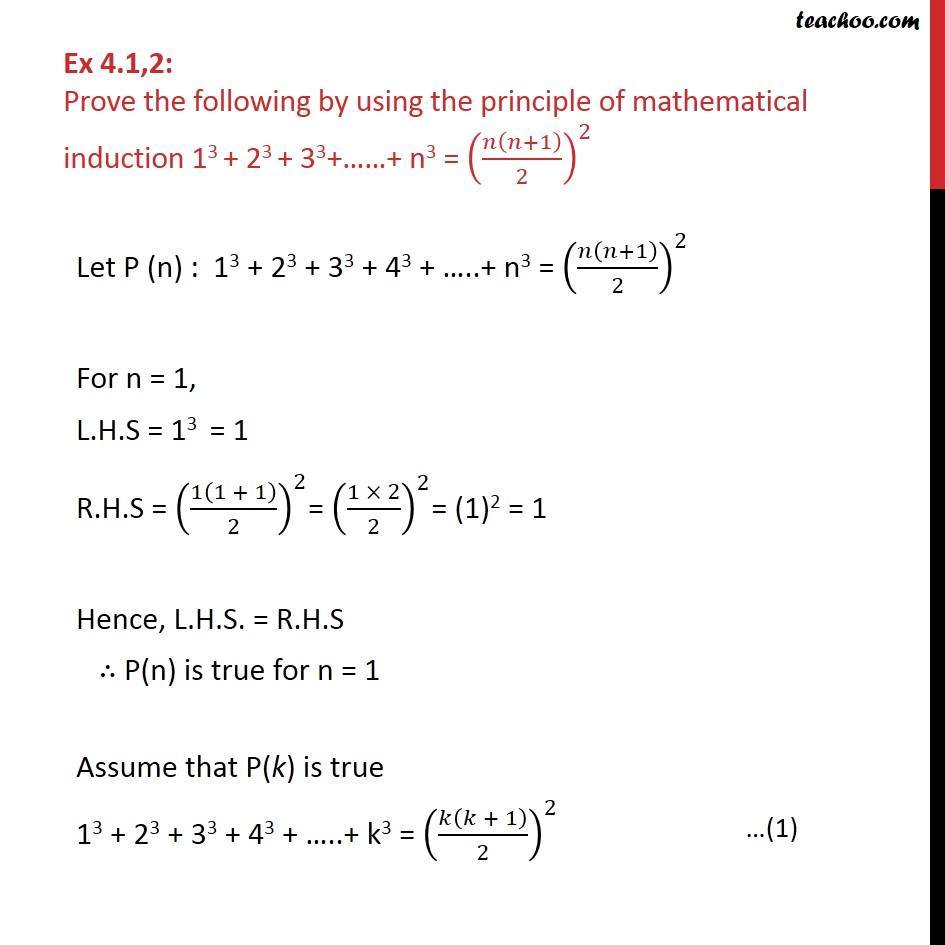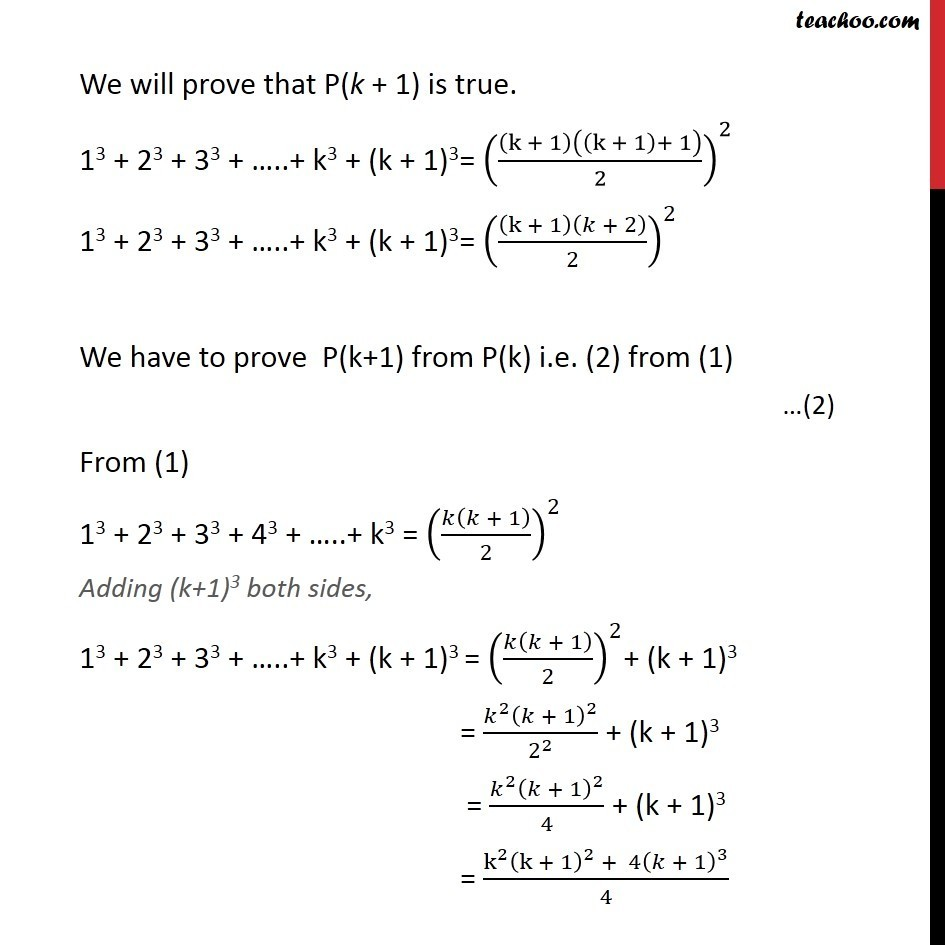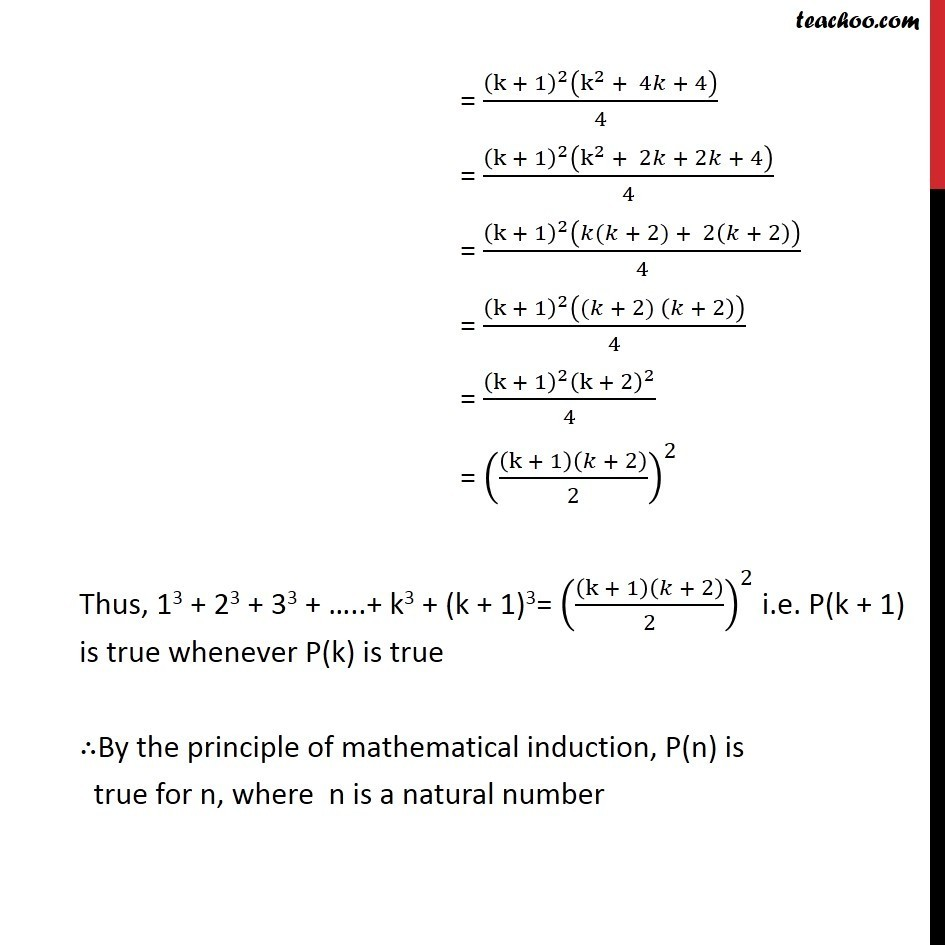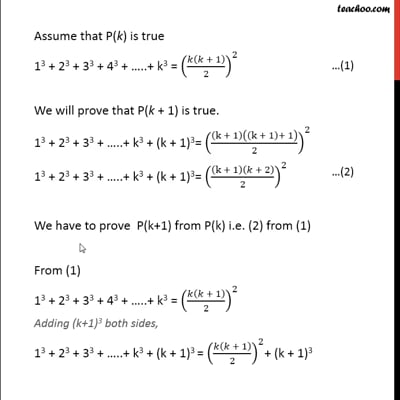Ex 4.1

Chapter 4 Class 11 Mathematical Induction (Deleted)
Serial order wiseThis video is only available for Teachoo black users

### Transcript

Ex 4.1,2: Prove the following by using the principle of mathematical induction 13 + 23 + 33+ + n3 = ( ( +1)/2)^2 Let P (n) : 13 + 23 + 33 + 43 + ..+ n3 = ( ( +1)/2)^2 For n = 1, L.H.S = 13 = 1 R.H.S = (1(1 + 1)/2)^2= ((1 2)/2)^2= (1)2 = 1 Hence, L.H.S. = R.H.S P(n) is true for n = 1 Assume that P(k) is true 13 + 23 + 33 + 43 + ..+ k3 = ( ( + 1)/2)^2 We will prove that P(k + 1) is true. 13 + 23 + 33 + ..+ k3 + (k + 1)3= ((k + 1)((k + 1)+ 1)/2)^2 13 + 23 + 33 + ..+ k3 + (k + 1)3= ((k + 1)( + 2)/2)^2 We have to prove P(k+1) from P(k) i.e. (2) from (1) From (1) 13 + 23 + 33 + 43 + ..+ k3 = ( ( + 1)/2)^2 Adding (k+1)3 both sides, 13 + 23 + 33 + ..+ k3 + (k + 1)3 = ( ( + 1)/2)^2+ (k + 1)3 = ( ^2 ( + 1)^2)/2^2 + (k + 1)3 = ( ^2 ( + 1)^2)/4 + (k + 1)3 = (k^2 (k + 1)^2 + 4( + 1)^3)/4 = ((k + 1)^2 (k^2 + 4 + 4))/4 = ((k + 1)^2 (k^2 + 2 + 2 + 4))/4 = ((k + 1)^2 ( ( + 2) + 2( + 2)))/4 = ((k + 1)^2 (( + 2) ( + 2)))/4 = ((k + 1)^2 (k + 2)^2)/4 = ((k + 1)( + 2)/2)^2 Thus, 13 + 23 + 33 + ..+ k3 + (k + 1)3= ((k + 1)( + 2)/2)^2 i.e. P(k + 1) is true whenever P(k) is true By the principle of mathematical induction, P(n) is true for n, where n is a natural number